Home > INT3 > Chapter 11 > Lesson 11.2.2 > Problem11-73

11-73.
1. 11-73.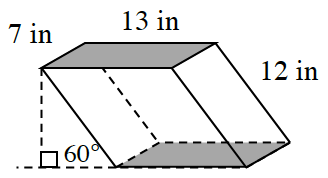Elliot has a modern fish tank that is in the shape of an oblique rectangular prism, shown at right. Homework Help ✎

1. Calculate the volume of water the tank can hold.

2. If Elliot has 25 fish, how crowded are the fish? That is, what is the density of fish measured in number of fish per cubic inch?

3. What is the density of fish in Elliot’s tank in fish per cubic foot?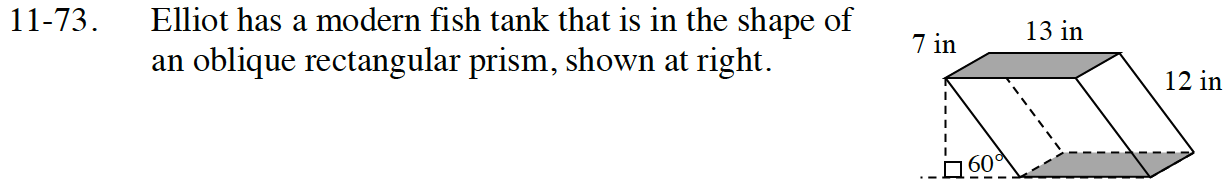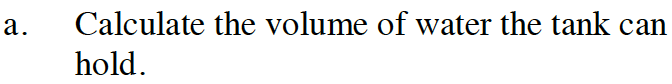Use a special right triangle to find the height of the parallelogram.

Find the height of the parallelogram:

linear scale factor = 6

$h=6(1\sqrt{3})=6\sqrt{3}$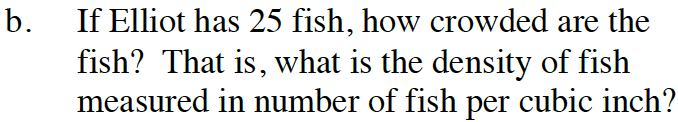$\frac{\text{number of fish}}{\text{number of cubic inches}} =$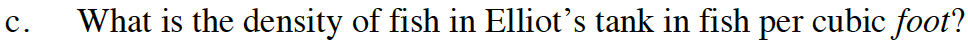Use dimensional analysis to convert

$\frac{\text{number of fish}}{\text{in}^3} \text{ into } \frac{\text{number of fish}}{\text{ft}^3}$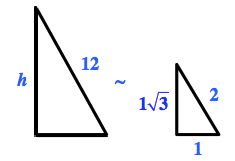Find the volume:

$V = 7 \cdot 13 \cdot 6\sqrt{3} = 546\sqrt{3}$

$\approx 945.7\: \text{in.}^3$